Hello,

In this tutorial-based article, we are going to learn about pH meters using pH sensors and Arduino.

## What is pH?

####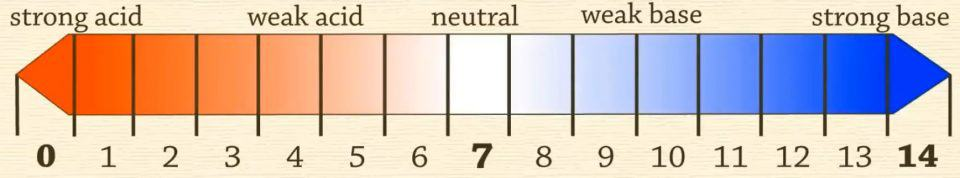• pH is a scale that is used to measure the acidity or basicity of an aqueous solution. The pH scale ranges from 0 to 14. 0 being the most acidic and 14 being the most basic. Pure water is neutral and thus has a PH of 7.

• pH is the logarithmic scale and it inversely indicates the concentration of hydrogen ions in an aqueous solution.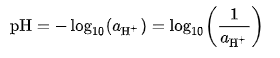• The above mentioned is the formula to calculate the pH of an aqueous solution.

## pH meter construction and working principle

####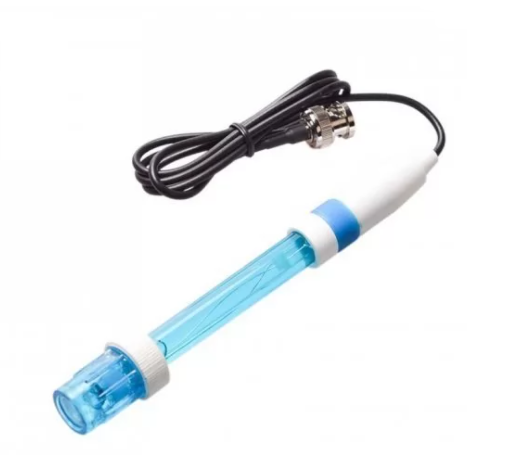• The pH sensor is a cylindrical glass structure that has a bulb situated at the bottom. This bulb is specifically designed to measure hydrogen-ion concentration when dipped in any solution.

• The hydrogen ions exchange the positively charged ions which are already situated inside the bulb, creating an electrochemical difference.

• This difference is interpreted by the electronic amplifier situated on the top. It further converts this difference into pH units.

• The pH meter sends the final pH values to the Arduino board.

• The Arduino receives those signals and sends the appropriate data to be displayed on the OLED screen.

• The values at first might not be accurate as the pH meter needs to be in the solution for a while to have the proper pH values.

# Circuit Diagram

####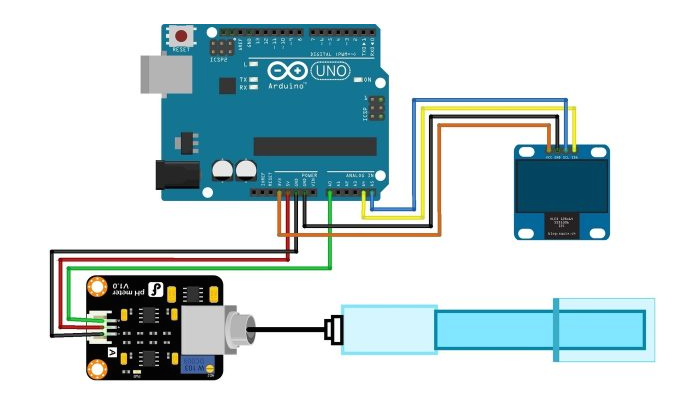• The pin connections for the circuit diagram are mentioned in the table below:
 Pins on Arduino Pins on pH sensor 5V VCC GND GND A0 Signal pin
 Pins on Arduino Pins on OLED Display 3.3V VCC GND GND A4 SDA A5 SCL

## Arduino Code

• Copy the Arduino code and upload it to your board via Arduino IDE.

## Testing the code

• We took 4 different solutions and tested the pH meter with them

####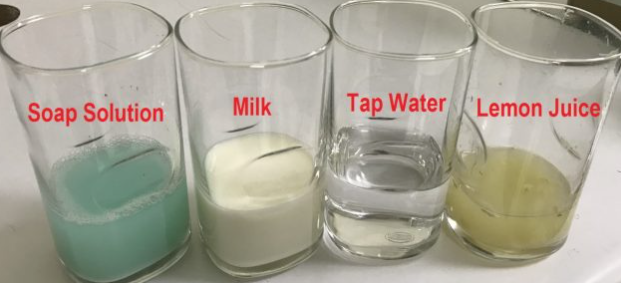• Testing it with highly acidic lemon water.